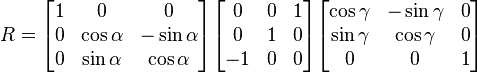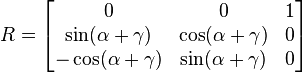# 四元数介绍

• 矩阵旋转
• 优点：
• 旋转轴可以是任意向量；
• 缺点：
• 旋转其实只需要知道一个向量+一个角度，一共4个值的信息，但矩阵法却使用了16个元素；
• 而且在做乘法操作时也会增加计算量，造成了空间和时间上的一些浪费；

• 欧拉旋转
• 优点：
• 很容易理解，形象直观；
• 表示更方便，只需要3个值（分别对应x、y、z轴的旋转角度）；但按我的理解，它还是转换到了3个3*3的矩阵做变换，效率不如四元数；
• 缺点：
• 之前提到过这种方法是要按照一个固定的坐标轴的顺序旋转的，因此不同的顺序会造成不同的结果；
• 会造成万向节锁（Gimbal Lock）的现象。这种现象的发生就是由于上述固定坐标轴旋转顺序造成的。理论上，欧拉旋转可以靠这种顺序让一个物体指到任何一个想要的方向，但如果在旋转中不幸让某些坐标轴重合了就会发生万向节锁，这时就会丢失一个方向上的旋转能力，也就是说在这种状态下我们无论怎么旋转（当然还是要原先的顺序）都不可能得到某些想要的旋转效果，除非我们打破原先的旋转顺序或者同时旋转3个坐标轴。这里有个视频可以直观的理解下；
• 由于万向节锁的存在，欧拉旋转无法实现球面平滑插值；

• 四元数旋转
• 优点：
• 可以避免万向节锁现象；
• 只需要一个4维的四元数就可以执行绕任意过原点的向量的旋转，方便快捷，在某些实现下比旋转矩阵效率更高；
• 可以提供平滑插值；
• 缺点：
• 比欧拉旋转稍微复杂了一点点，因为多了一个维度；
• 理解更困难，不直观；

# 四元数和欧拉角

## 基础知识

p=qpq1

N
(q)
=1，即q1=q。右边表达式包含了四元数乘法。相关的定义如下：
• 四元数乘法：q1q2=(v1×v2+w1v2+w2v1,w1w2v1v2)

• 共轭四元数：q=(v⃗ ,w)

• 四元数的模：N(q) = √(x^2 + y^2 + z^2 +w^2)，即四元数到原点的距离

• 四元数的逆q1=qN(q)

• 用于旋转的四元数，每个分量的范围都在（-1，1）；

• 每一次旋转实际上需要两个四元数的参与，即q和q*；

• 所有用于旋转的四元数都是单位四元数，即它们的模是1；

• 实际上，在Unity里即便你不知道上述公式和变换也丝毫不妨碍我们使用四元数，但是有一点要提醒你，除非你对四元数非常了解，那么不要直接对它们进行赋值

• 如果你不想知道原理，只想在Unity里找到对应的函数来进行四元数变换，那么你可以使用这两个函数：Quaternion.EulerQuaternion.eulerAngles。它们基本可以满足绝大多数的四元数旋转变换。

## 和其他类型的转换

q=((x,y,z)sinθ2cosθ2)

x = sin(Y/2)sin(Z/2)cos(X/2)+cos(Y/2)cos(Z/2)sin(X/2)
y = sin(Y/2)cos(Z/2)cos(X/2)+cos(Y/2)sin(Z/2)sin(X/2)
z = cos(Y/2)sin(Z/2)cos(X/2)-sin(Y/2)cos(Z/2)sin(X/2)
w = cos(Y/2)cos(Z/2)cos(X/2)-sin(Y/2)sin(Z/2)sin(X/2)
q = ((x, y, z), w)

# 四元数的插值

Q3  = (sin((1-t)A)/sin(A))*Q1 + (sin((tA)/sin(A))*Q2)
Q = Q3/|Q3|，即单位化

# 四元数的创建

Vector3 newVector = Quaternion.AngleAxis(90, Vector3.up) * Quaternion.LookRotation(someDirection) * someVector;

transform.rotation = Quaternion.AngleAxis(degrees, transform.right) * transform.rotation;

# 补充：欧拉旋转

## 欧拉旋转是怎么运作的

1. 绕坐标系E下的Z轴旋转α，绕坐标系E下的Y轴旋转β，绕坐标系E下的X轴旋转r，即进行一次旋转时不一起旋转当前坐标系；
2. 绕坐标系E下的Z轴旋转α，绕坐标系E在绕Z轴旋转α后的新坐标系E'下的Y轴旋转β，绕坐标系E'在绕Y轴旋转β后的新坐标系E''下的X轴旋转r， 即在旋转时，把坐标系一起转动；

transform.Rotate(new Vector3(0, 30, 90));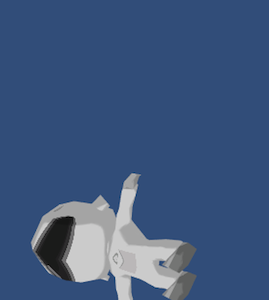// First case
transform.Rotate(new Vector3(0, 30, 0));
transform.Rotate(new Vector3(0, 0, 90));

// Second case
//		transform.Rotate(new Vector3(0, 0, 90));
//		transform.Rotate(new Vector3(0, 30, 0));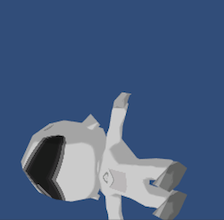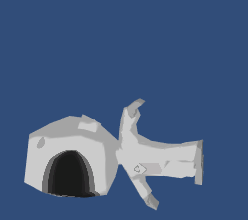## 数学模型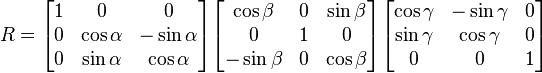## 万向节锁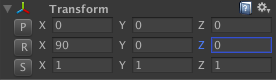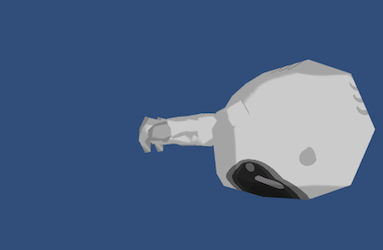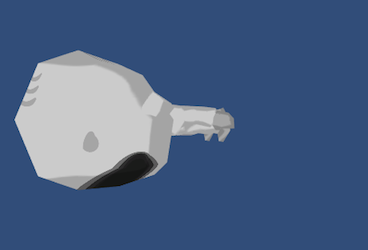transform.Rotate(new Vector3(0, 0, 40));
transform.Rotate(new Vector3(0, 90, 0));
transform.Rotate(new Vector3(80, 0, 0));`

## 数学解释